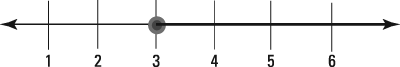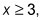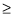##### Praxis Elementary Education For Dummies with Online Practice TestsThe Math section of the Praxis Elementary Education exam may contain a question that requires you to graph the solution to an inequality on a number line.

To begin, you put a dot on the number in the solution. If the symbol is > or <, then "or equal to" is not part of the solution, so you use a hollow dot to show that the number is just the boundary of a range of numbers and is not part of it.

"or equal to" is part of the solution, so you put a darkened in dot on the number to show that it is included in the range of numbers. Suppose you want to graph the solution to the inequality x > 4. Put a hollow dot on 4 on the number line, and then shade the number line in the direction that includes all numbers greater than 4, as shown here.

## Practice question

1. Given the following graph,which of the following inequalities has a solution that is represented by the graph?

1. The correct answer is Choice (B). The graph representswhich is the solution to Choice (B) and none of the other choices. A solid filled in circle on a number line represents including the corresponding number. Sincemeans "greater than or equal to," the 3 is included. Thus, the interior of the circle is darkened. The line is darkened to the right of 3 because the graph represents 3 and all numbers that are greater.